UTS Prototyping Physical Interaction — Spring 2017 - this is a reblog of a uni. assignment.

## Design context

Develop an element of an interactive system using one or more sensors.

What I've developed: a UI element (rendered to an LED matrix) that moves left and right according to the orientation of the Pi. I developed a Pong clone for the Pi in which this element was employed as a paddle.

Rationale: I wanted to develop something fun and personally challenging to implement. The LED matrix provides a retro aesthetic, which you wouldn't get otherwise on a smartphone screen. Conversely, the gyroscope is a relatively modern addition in the design of technology (your gameboy didn't have one), and makes interaction much more intuitive. Given these two components, it was evident that recreating something akin to Pong would be an interesting and exemplary interactive system.

### Types of contexts

This element tracks the orientation of the Raspberry Pi (as an angle of 360 degrees) but notably only uses a smaller range of 52 degrees.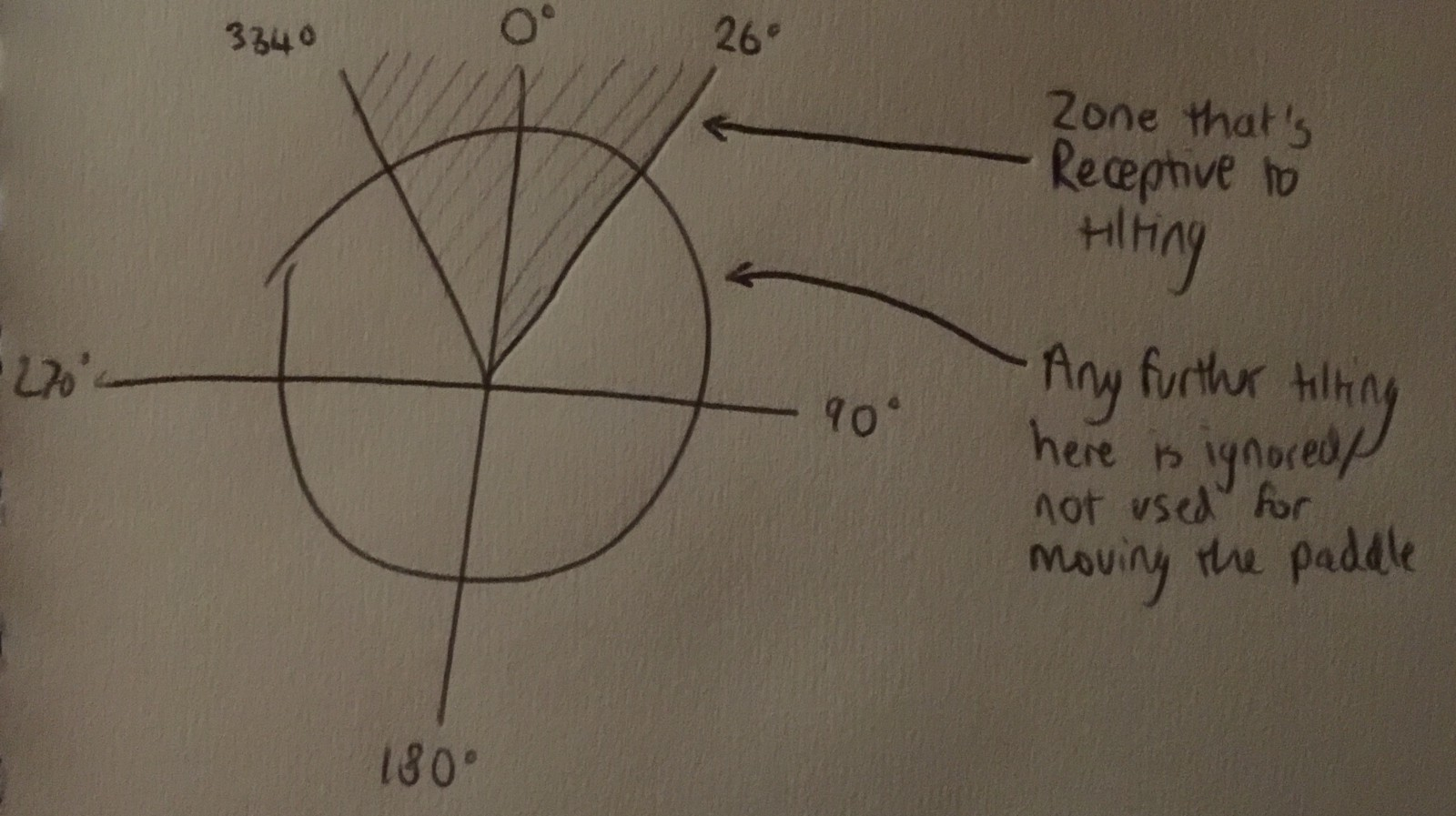The rationale for this is because the display is needed to convey information, the range for tilting is restricted to what keeps it in view.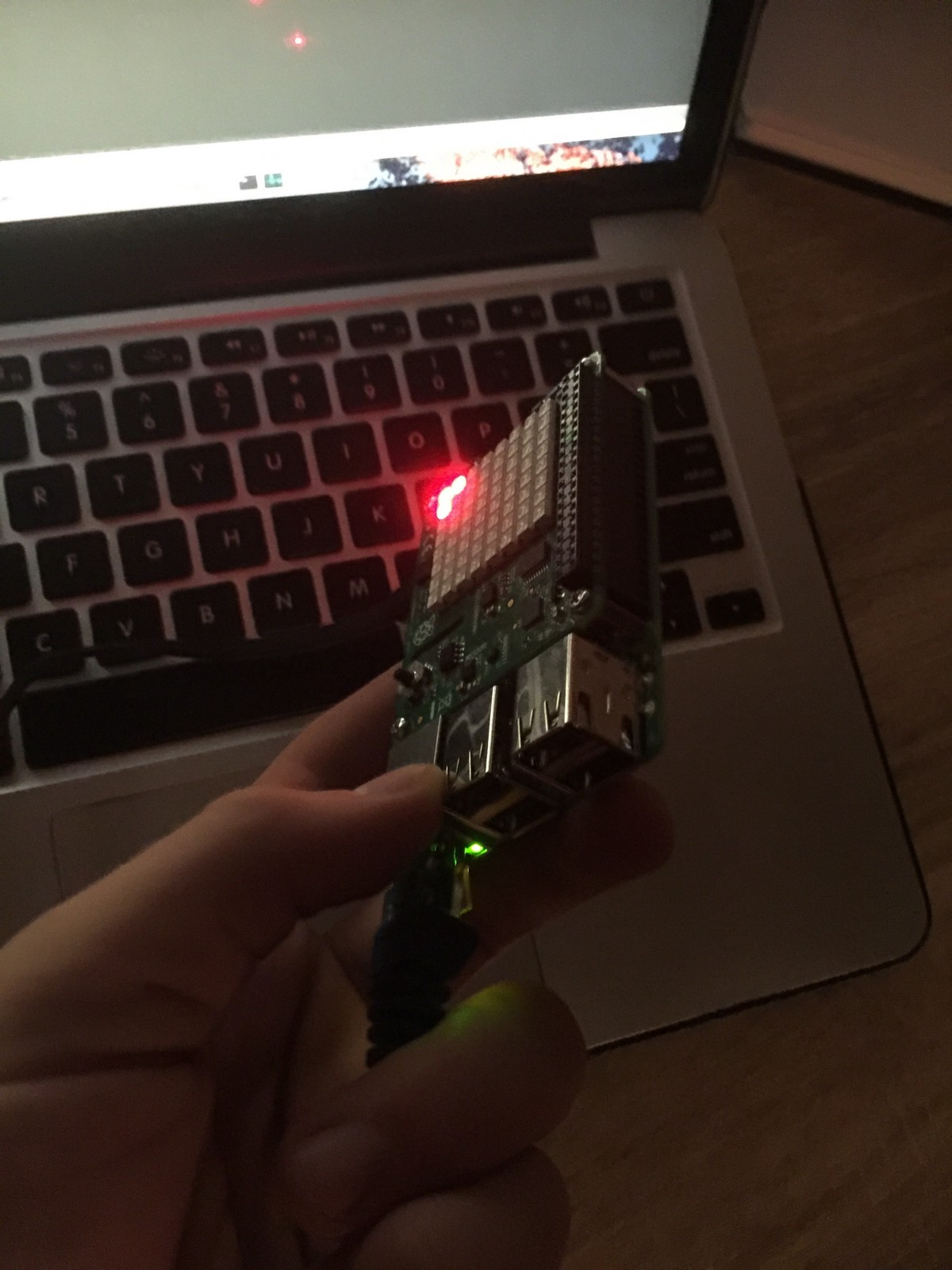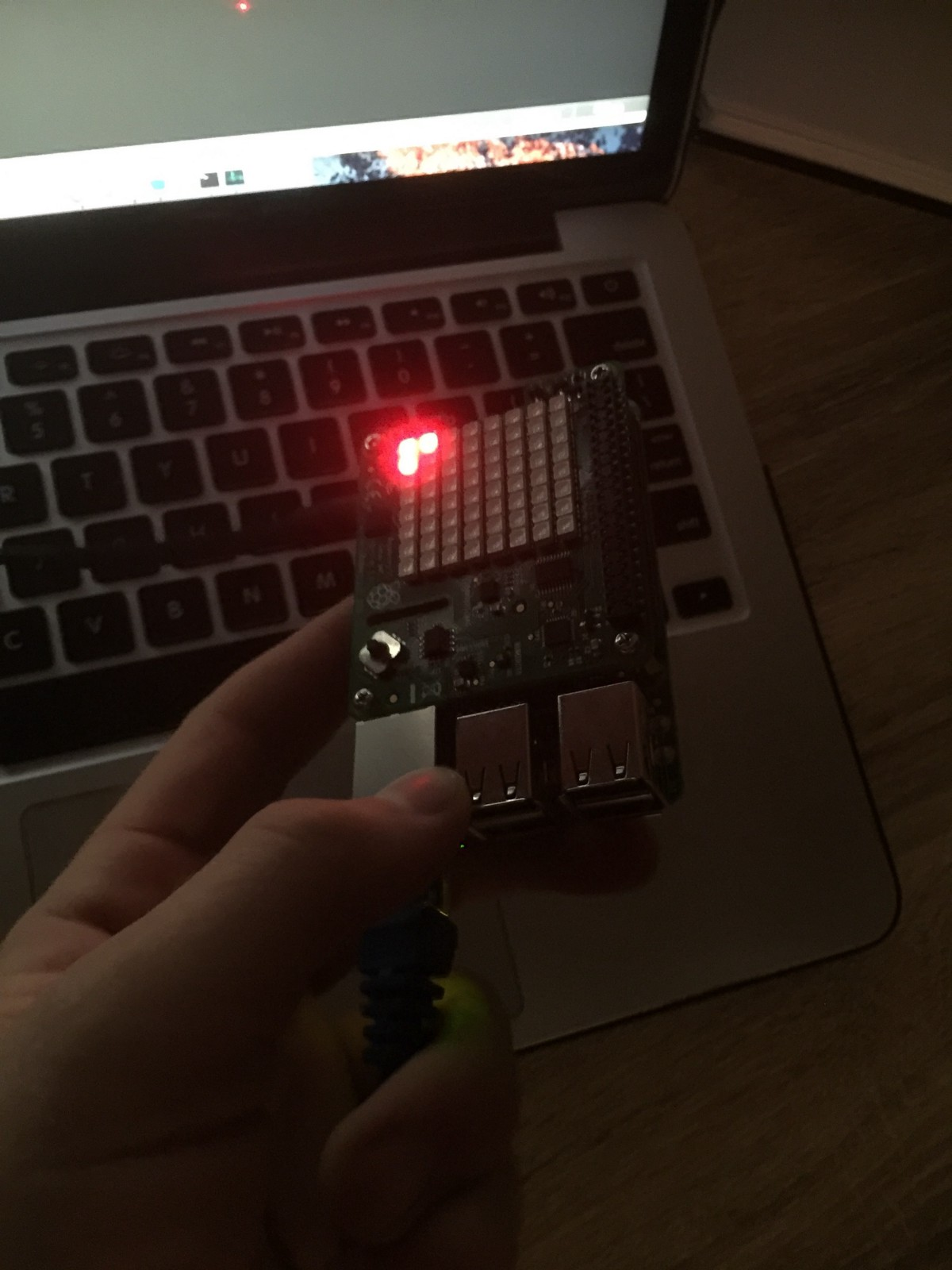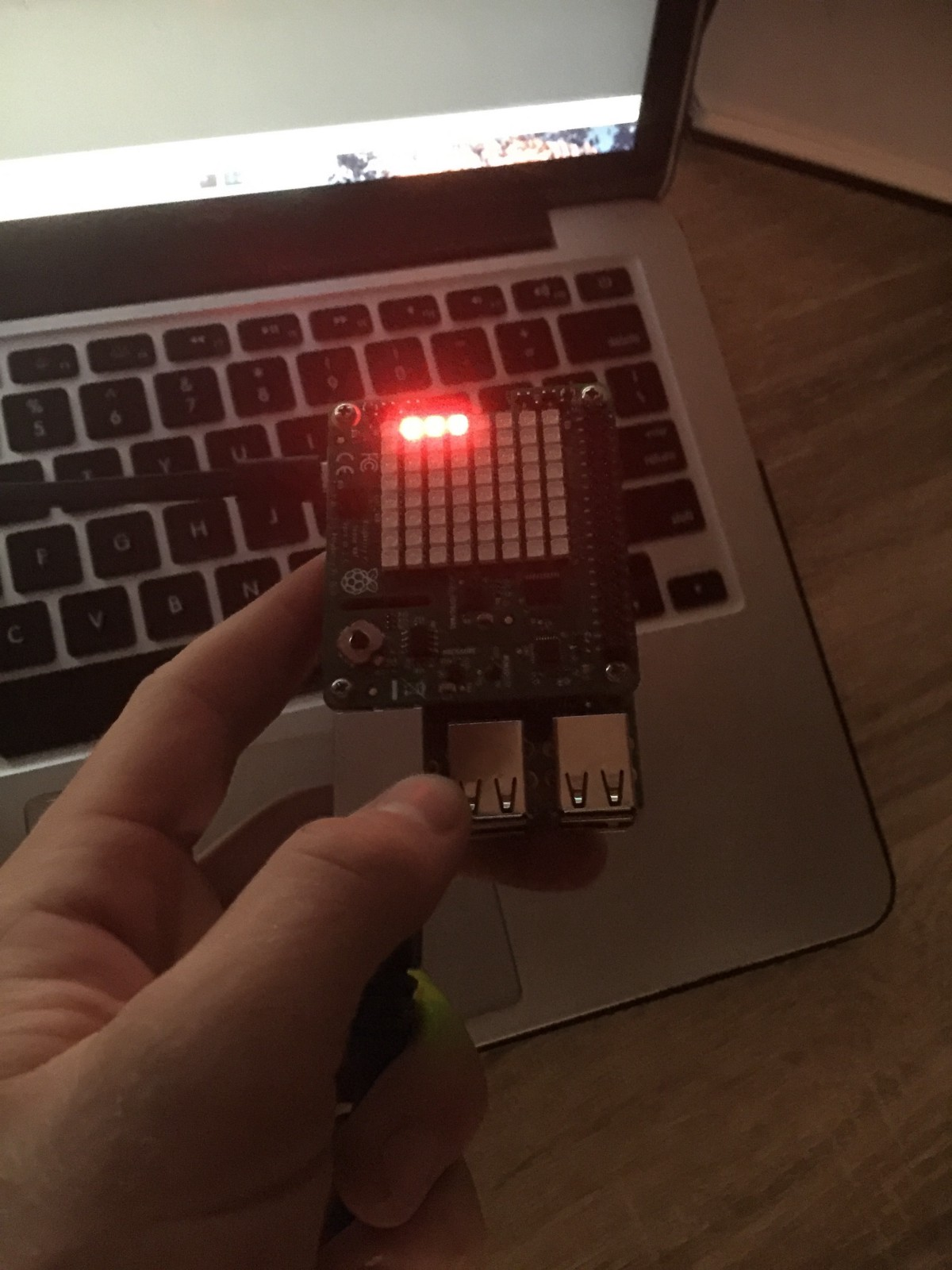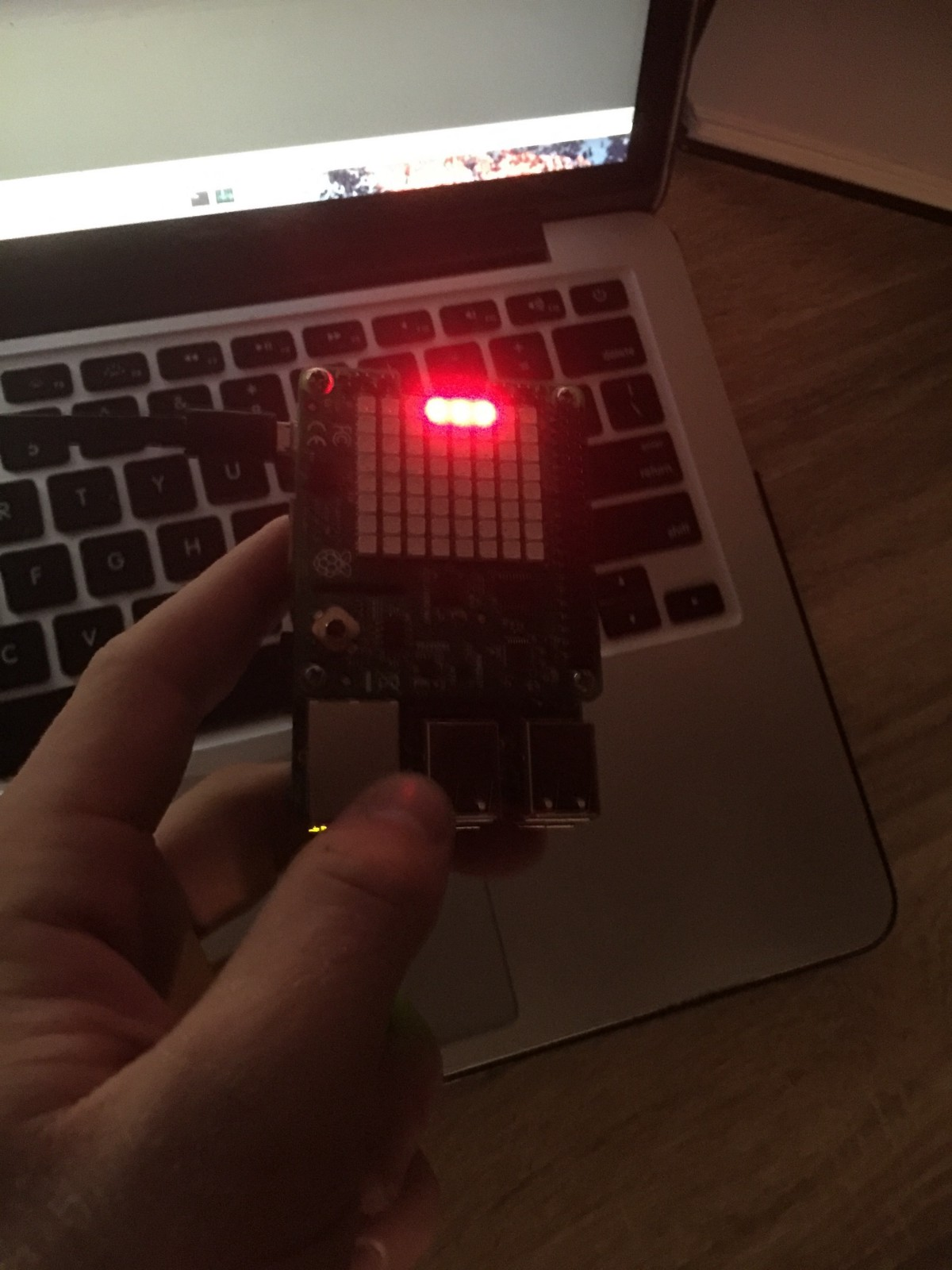Tilting in this range works (because you can see the screen!).

Some potential contexts this could be used in:

• Presenting the user with a binary choice to make, and use the tilt to determine yes (tilt left) or no (tilt right), where the LED display provides visual feedback on the outcome.
• In combination with another element (such as a button) as a control with a spectrum of values e.g. using it as a volume slider
• With an LED display to provide an intuitive gameplay element (described below)

## Demo - Pong

Things you will need:

• Raspberry Pi and its OS on the microSD card
• Raspberry Pi Sense HAT
• A method to connect to the Pi to upload and run code (HDMI+keyboard, Ehternet+SSH, etc.)

How to use it:

1. Open a terminal to your Pi
2. Run nano pong.py
3. Copy and paste my code below, and hit Ctrl+O to save and exit.
from __future__ import division
from sense_hat import SenseHat
import time

sense = SenseHat()

running = True
angle_length = 26

def min(a,b):
if a < b:
return a
return b

def __init__(self):
self.angle = 0.0

def get_pos(self):
return (
)

def update(self, orientation):
angle = orientation

if angle < 26:
angle = 0.0 + (26-angle)
elif (360 - angle_length) < angle < 360:
angle = 26.0 + (360 - angle)
else:
# if min(360 - angle, 0) == angle:
if min(angle, 180) == angle:
angle = 0.0
else:
angle = 52.0

angle = (angle / 52.0) * 7
# for some reason, I have the inverse behaviour
angle = int(7 - angle)

if (angle - 1) < 0:
self.paddle_pos_right = [0, angle + 1]
elif (angle + 1) > 7:
self.paddle_pos_left = [0, angle - 1]
else:
self.paddle_pos_left = [0, angle - 1]
self.paddle_pos_right = [0, angle + 1]

def render(self):

X = 0
Y = 1

class Ball():
def __init__(self):
self.pos = [6, 3]
self.direction = -1
self.angle = 0 # -1, 0, 1
pass

collide = False

if self.pos[X] == 7:
collide = True
elif self.pos[X] == 0:
collide = True

if self.pos[Y] == 0:
collide = True
self.angle = 1
if self.pos[Y] == 7:
collide = True
self.angle = -1

if not collide:
if pos[X] == self.pos[X]-1 and pos[Y] == self.pos[Y]:
collide = True
break

if collide:
self.direction *= -1
self.angle *= self.direction

self.pos[X] += self.direction * 1
self.pos[Y] += self.angle * 1

def render(self):
sense.set_pixel(self.pos, self.pos, 0, 0, 255)

ball = Ball()

while running:
time.sleep(0.05)

roll = sense.get_orientation_degrees()['roll']

1. Run python pong.py. Turn the board from side to side to move your paddle. The ball will bounce around. When it hits the paddle it will bounce back.